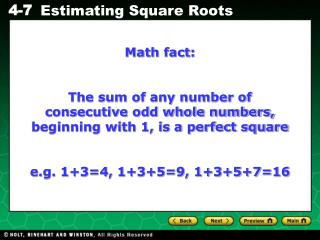# Math fact: - PowerPoint PPT PresentationDownload PresentationMath fact:

Math fact:Download Presentation## Math fact:

- - - - - - - - - - - - - - - - - - - - - - - - - - - E N D - - - - - - - - - - - - - - - - - - - - - - - - - - -
##### Presentation Transcript

1. Math fact: The sum of any number of consecutive odd whole numbers, beginning with 1, is a perfect square e.g. 1+3=4, 1+3+5=9, 1+3+5+7=16

2. 55 55 is between 7 and 8 because 55 is between 49 and 64. 49 < 55 < 64 7 < 55 < 8 Example 1: Estimating Square Roots of Numbers The 55 is between two integers. Name the integers. Explain your answer. 36, 49, 64, 81 List perfect squares near 55. Find the perfect squares nearest 55. 49 < 55 < 64 Find the square roots of the perfect squares.

3. Approximate √135 to the nearest hundredth. √ √121 < 144 √135 < 11 < 135 < 12 √ Example 2: Approximating Square Roots to the Nearest Hundredth Step 1 Find the value of the whole number. Find the perfect squares nearest 135. 121 < 135 < 144 Find the square roots of the perfect squares. The number will be between 11 and 12. The whole number part of the answer is 11.

4. Approximate √135 to the nearest hundredth. Example 2 Continued Step 2 Find the value of the decimal. Find the difference between the given number, 135, and the lower perfect square. 135 – 121 = 14 Find the difference between the greater perfect square and the lower perfect square. 144 – 121 = 23 1423 Write the difference as a ratio. Divide to find the approximate decimal value. 14 ÷ 23 ≈ 0.609

5. Approximate √135 to the nearest hundredth. The approximate value of 135 to the nearest hundredth is 11.61. Example 2 Continued Step 3 Find the approximate value. Combine the whole number and decimal. 11 + 0.609 = 11.609 Round to the nearest hundredth. 11.609 ≈ 11.61

6. 600 ≈ 24.494897… Example 3: Using a Calculator to Estimate the Value of a Square Root Use a calculator to find 600. Round to the nearest tenth. Use a calculator. Round to the nearest tenth. 600 ≈ 24.5 600 rounded to the nearest tenth is 24.5.